# Electric Field due to a disk of radius R in the xy-plane

neroE
Homework Statement:
N/A
Relevant Equations:
Coulomb's law mainly.
Hello,
This question, which I found in various electricitiy and magnetism books (e.g. Introduction to electrodynamics grif.).

There are many variations of this question, I am mainly interested in the following setup of it:
-Suppose there is a charged disk of radius R lying in the xy-plane, and the electric field is uniform. What is the electric field at the point P(0,0,z)?

I know this can be solved by first considering a ring, deriving a formula for it, and then summing the infinite number of rings that make up a disk (via integration), and thus, the desired result is yielded. However, I am more interested in how to approach this using surface integration?I started with the parametrization G(u,v) = <ucos(v),u*sin(v),0> and found the normal unit vector to be <0,0,1>
where: 0<u<R and 0<v<2*pi

But, I am not sure how to continue from there, I attached a pdf showing my subsequent steps, which I am not sure if it is valid or not.

#### Attachments

• 1.pdf
190.3 KB · Views: 3

Homework Helper
Hello @neroE ,##\qquad ## !​

which I found in various electricitiy and magnetism books
and the electric field is uniform
do you mean 'and the charge is uniformly distributed' ##\qquad## ?

You also want to
• explain the symbols you are using (##G## ?) in words or in a picture
• write legibly -- or, better: use ##\LaTeX##

##\ ##

•nasu, MatinSAR and neroE
neroE
Hi @BvU ,
Yes, sorry, the charge is uniformly distributed.
I did not use any symbols except the common three symbols:
r: radius that varies in the disk (I.e.starts from r=0 till r=R)
theta: which is the angle that kind of describes the ring/disk (I.e. starts from 0 to 2pi).
G: This is just to indicate a mapping is occuring (I.e. G(r,theta) means parametrizing the surface using r and theta, and then: G(r,theta) = <rcos(theta),rsin(theta),0> means x=rcos(theta), y=rsin(theta), z=0.
I apologise if this notation is not that common, I read it in a calculus textbook before.
Lastly, I know some latex; however, I may not be able to write the pdf I attached completely in latex as I did not learn that much latex. So, please excuse my first post not being in latex, and hopefully my next posts will be in Latex as I will be learning it.

Staff Emeritus
Homework Helper
Your expression ##dr\,d\theta## for ##dA## is wrong. It needs to have units of area. You forgot to multiply by the Jacobian, which will give you a factor of ##r##.

•MatinSAR, vanhees71 and neroE
Homework Helper
Ok, and I suppose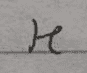is the Coulomb constant, a.k.a. ##\displaystyle {1\over 4\pi\varepsilon_0}##.

( re notation: I use separate symbols for the vector in the disk and the vector from ## dA## to point P )

You have ##\vec r = \vec p -\vec r' ## with ##\vec p =<0,0,z>## and ##\vec r'= <r'\cos\theta,r'\sin\theta,0>## and ##\hat r = {\vec r \over |\vec r|} ##
And then I can follow $$d\vec E = {1\over 4\pi\varepsilon_0} \; {1\over r^2}\;{\vec p - \vec r' \over |\vec r| } \; dQ$$
where ## dQ = \sigma dA = \sigma r' dr'\, d\theta ## (*)

Note that ##dQ## is a scalar !

( (*) I have the impression you overlooked the factor ##r'## ? Nice to see vela agrees...)​

In sort, I agree with your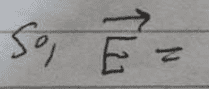(quoting ##\LaTeX## really is a lot more comfortable).

Except that it looks as if you think ##dA## is a vector and then in the subsequent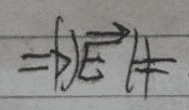you come up with dot product ##\vec r \cdot \vec n##

which I am not sure if it is valid or not
I don't think it's valid, but here it works because you pick out the ##z## component, which is the only one that doesn't cancel from symmetry.

##\ ##

•MatinSAR, neroE and vela
neroE
Hi,
I learnt some latex, not the best but at least better.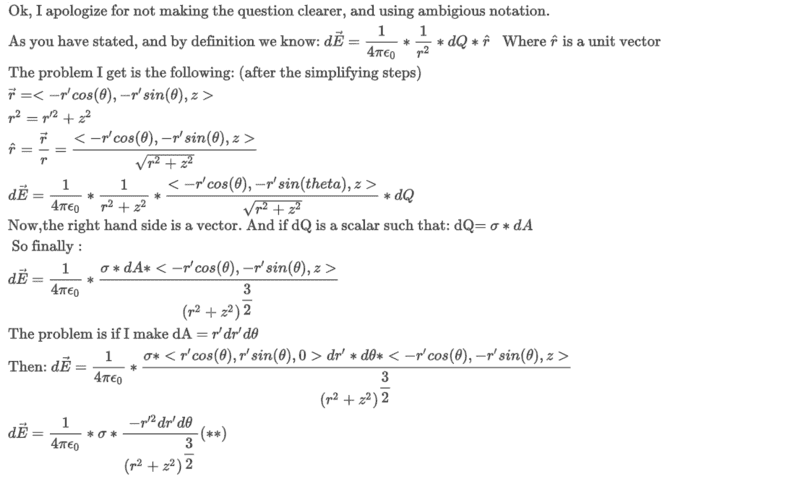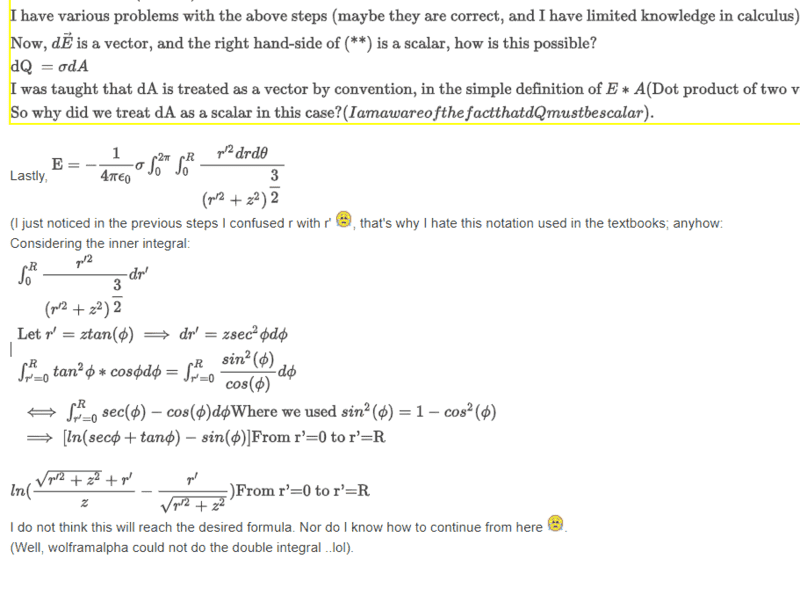Thanks @vela and @BvU.
I think my main initial problem was treating the problem as a vector surface integration question, and in this, we always convert F*dA(vector) to scalar surface integral by parametrization and finding the normal vector (which is kinda the "jacobian"). But, as both of you have stated, I just realized that it is just a double integration problem and no need for vector fields I guess.

Homework Helper
Hi,
I learnt some latex, not the best but at least better.

Great ! Would be even better to embed it in the post: in-line math enclosed in double ## and displayed math enclosed in double 
More tips: use \cos and \sin and ##\theta## instead of ##(\theta)##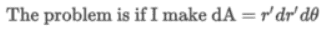I think my main initial problem was treating the problem as a vector surface integration question, and in this, we always convert F*dA(vector) to scalar surface integral by parametrization and finding the normal vector (which is kinda the "jacobian"). But, as both of you have stated, I just realized that it is just a double integration problem and no need for vector fields I guess.
Yep. As stated, ##dQ = \sigma \, dA = \sigma \,r'\,dr'\, d\theta## , ALL scalars. So you can't replace ##r'\ (=|r'|) ## by ## <r'\cos\theta,r'\sin\theta,0>##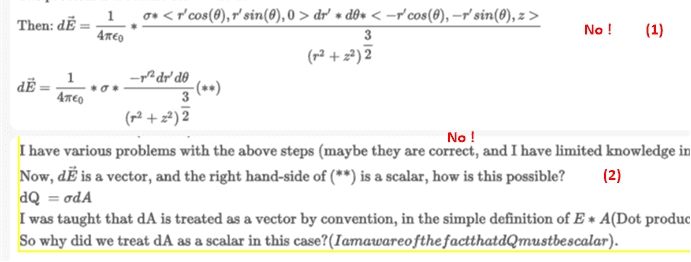(1) not correct to replace ##dA## by a vector
(2) you take a dot product where you should multiply with ##|r'|## (and in the denominator you write ##r^2## instead of ##r'^{\, 2} \ ##)

But I think we start repeating things.

##\ ##

••neroE, vanhees71 and MatinSAR
neroE
Alright, thank you very much @BvU
I learnt a lot of things from this thread & some latex.•BvU